## Texture Synthesis

CS 348B - Computer Graphics: Image Synthesis Techniques
Spring Quarter, 1997
(TA) Lucas Pereira
Lecture notes for Tuesday, April 29
Handout #20

## Texture Synthesis

### Contents:

• Review: Texture framework
• Types of Texture
• Scanned Texture
• Synthesized Texture
• To Store or Compute?
• Perlin's Image Synthesizer ('85)
• Worley's Cellular Texture Basis Function ('96)
• Heeger's Pyramid-Based Texture Analysis/Synthesis ('95)
• Turk's Reaction-Diffusion ('91)
• Dorsey's Flow and Changes in Appearance ('96)
• Fleischer's Cellular Texture ('95)

### Review: Texture framework

• What is Texture?
• color (Primary focus today)
• normal (bump map)
• position (displacement map)
• shininess
• transparency
• micro-geometry (such as Fleischer, below)
• ...
• How is it parameterized?
• 2D
• 3D
• 4D (view-dependent texture -- similar to a lumigraph, or a geometry-corrected light field)
• 5D (volumetric view-dependent texture)
• 6D (spatially varying BRDF)
• time
• wavelength
• Mapping to the surface
• 2D: parameterize (warp) onto the surface
• 3D: No warp, surface cuts through 3D volume

### Types of Texture:

• Scanned Texture
• From real life
• Sampled, stored, resampled
• Synthesized Texture (Today's topic)
• Equation-based
• f(u,v) or f(x,y,z)
e.g. linear ramp, checkerboard, boolean functions, polynomials, sine waves, ...
• Random
• Fractal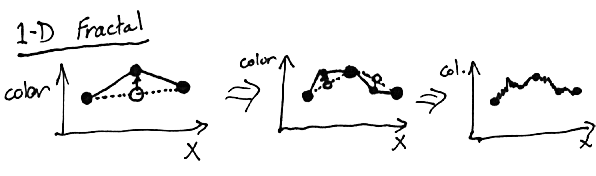• Perlin ('85)
• Worley ('96)
• ...
• Statistics-based
• Heeger ('95)
• ...
• Procedure-based (Simulation-based)
• Reaction-Diffusion (Turk '91)
• Cellular Texture Generation (Fleischer '95)
• Flow (Dorsey '96)

### Store or Compute?

• Compute on the Fly
• Compute function for every sample
• Very little memory
• Need to have consistent mapping
(If random, need to use same random seed each time)
• Intermediate Storage
• Resampling issues
• Lots of memory?
• One-time generation cost -- can be expensive
• Reaction Diffusion
• Heeger
• Multi-step algorithms (my crystal)
• Tiling
• Can save memory
• Can introduce visible repetition

### An Image Synthesizer Ken Perlin Siggraph 1985

PSE: "Pixel Stream Editor"
• Inspired by Cook's Shade Trees (Siggraph '84)
• High-level language for describing shading function at each pixel
• Input variables: surface, point, normal, etc.
• Stochastic functions: Noise, DNoise, Turbulence ...
• Defined over 3D space
• Noise: narrow band of frequencies
• eg: grid, each lattice-point with random number
• Tri-linearly interpolate between points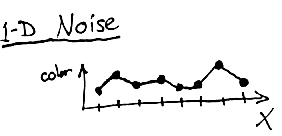• DNoise: vector gradient of Noise
• Turbulence: wide range of frequencies
• ```	function turbulence(p)
t=0
scale=1
while (scale > pixelsize)
t+= abs(Noise(p/scale)*scale)
scale /= 2
return t
```
• definition self-similar (fractal)
• abs causes discontinuous boundaries at all scales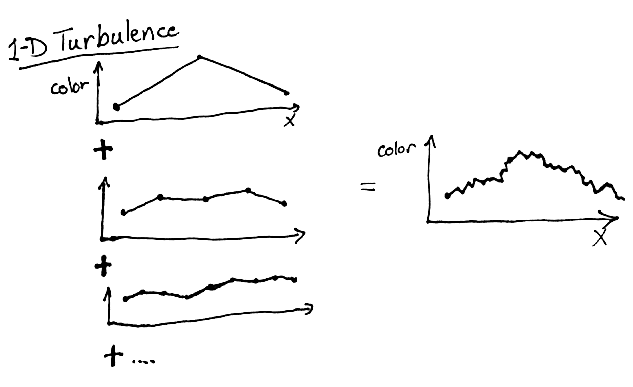• Example:
```	color = white * Noise(point)
```
• Fancier example:
```	function boring_marble(point)
x = point
return marble_color(sin(x))
```
• Even fancier example: (EFE)
```	function marble(point)
x = point + turbulence(point)
return marble_color(sin(x))
```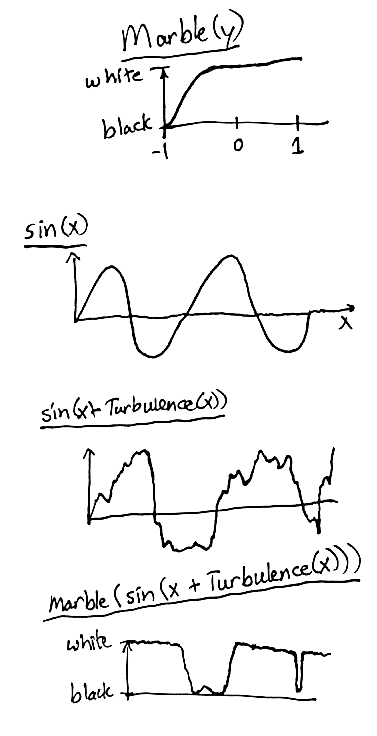• This used for his marble vase

### A Cellular Texture Basis Function Steven Worley Siggraph 1996

• Similar to Perlin, except adds new functions:
• distributes pseudo-random points through space
• F1 = distance to closest point
• slope discontinuity equivalent to Voronoi diagram
• F2 = distance to 2nd closest point
• F3 = distance to 3rd closest ...
• can do polka-dots, flagstone ....
• fractal version (multiple levels of resolution, like Perlin) can have detail at a wide range of scales

### Pyramid-Based Texture Analysis/Synthesis David Heeger & James Bergen Siggraph 1995

• mainly 2D, non-directional 3D
• Builds statistics about a given texture (often scanned)
• Manipulates noise image to have same statistics
• Histograms of frequency content for each direction, frequency band
• Why create new texture?
• Can be arbitrarily large
• Can be seamlessly tileable
• Works well for noisy textures with non-distinguishable features.
• Fails for distinguishable features, and correlation of high frequency over long distances, such as edges
• Steerable filters
• A convolution kernel that picks up a certain frequency band in a certain direction
• Analyzing phase
• We filter original image with a bank of these steerable filters, getting a pyramid of images of each frequency/direction
• We build histograms of pixel intensities in each image
• Histogram-matching phase
• We filter noise image with the same bank of these steerable filters, getting a pyramid of images of each frequency/direction
• We build histograms of pixel intensities in each image
• Compute a scaling function of pixel values for each of the images in the noise pyramid, so that it has the same histogram as corresponding source pyramid image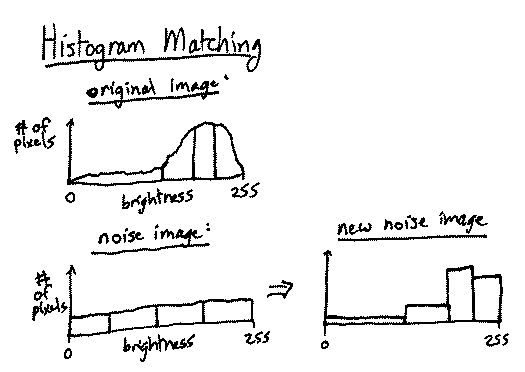• Collapse noise pyramid
• filters not entirely indepent
• This alters histograms
• repeat Analyze-Match about 5 times

### Generating Textures on Arbitrary Surfaces Using Reaction-Diffusion Greg Turk Siggraph 1991

• Simulates the interaction of 2 (or more) chemicals
They interact until they form a stable state
• Similar to how the embryo of a worm forms segments
• da/dt = F(a,b) + Da * Grad(a)^2
• db/dt = G(a,b) + Db * Grad(b)^2
• G(a,b) is the reaction between chemicals a, b
• Db is the diffusion constant for b
• Grad(b)^2 is local gradient of b's concentration, which affects its diffusion speed
• Map chemical concentrations to a texture (color, bump, etc)

### Flow and Changes in Appearance Julie Dorsey et. al. Siggraph 1996

• 2D
• Simulate water flow as a particle system
• factors: gravity, friction, wind, roughness ...
• parameters:
• rendering: diffuse color, specular color, shininess
• simulation: roughness, absorption, absorptivity
• deposits: diffuse color, adhesion rate, solubility rate
• Rendering: Deposits alpha blended over surface

### Cellular Texture Generation Kurt W Fleischer et. al. Siggraph 1995

• 2D
• Cellular particle simulator
• Uses differential equations to place the cells
• Constraints specified as energy functions to be minimized, e.g.:
• Go to the object surface
• Die if too far from surface
• Align an axis with a vector field